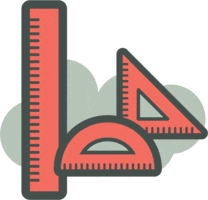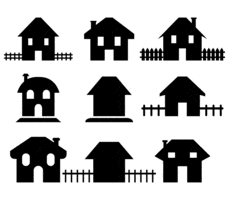# Math Worksheets Land

Math Worksheets For All Ages

# Math Worksheets Land

Math Worksheets For All Ages

Home > Topics >

# Trigonometry Worksheets

Triangles have a very unique relationship between their sides and angles. That is why a complete branch of mathematics named trigonometry studies the geometry of triangles. You can use this math to learn many different things about a triangular shape when you have little information on it. Even though trig is underrepresented in the Core Curriculum I thought it to be an important part to the basic math curriculum. I find that Trig makes an appearance in High School Functions and Geometry, but that is about the limit of where it goes. This series of worksheet topics and lessons will help you better understand how to approach this form of mathematics and make sense of it all.

### What is Trigonometry?Like it or not, at some point, you are going to study this branch of mathematics. Where most of us might think of trigonometry as a complicated concept of advanced mathematics, a firm grasp on basics can go a long way. It is basically triangle geometry. The entire focus of study is understanding the relationships between sides and angles of triangles. Triangles are a particularly unique geometric shape because the sum of all the interior angles is always constant and if it contains a right angle, we can delineate the sides and other angles with easy.

Before we jump on to explaining some basic concepts, let us first understand trigonometry with its basic definition. Trigonometry is one of the major concepts that students need to master. It is a blend of geometry and mathematical calculations. When we study trigonometry, we are mainly concerned with the relationships between sides and angles of a triangle.

But don't let 'tri' in trigonometry confuse you. Trigonometry mainly deals with triangles, but it is not limited to triangles. Many geometrical shapes can easily be broken down into triangles and solved by using trigonometry. So, trigonometry can help resolve many other shapes. We have different trigonometric formulae and ratios for figuring out the measurements of angles and sides of triangles.

Basic trigonometric definitions - Some of the basic trigonometric definitions are:

Hypotenuse- it is the longest side of a right-angle triangle, and the side lies opposite to the right angle.

Opposite- it is the side across from the angle under consideration. Opposite doesn't intersect on the vertex of the angle.

Adjacent- it is the side next to the angle under consideration. Or it is the side other than the hypotenuse that is included in the vertex.

### How is Trigonometry Used in The Real World?There are seriously too many real-world applications to list. This form of math helps us model just about any situation that involves a triangular geometric shape. The obvious application is found in architecture. If you look at many different forms of construction, you will see it evidently used. A triangle forms a strong structure when formed with materials. Look at the shape of roofs of many free-standing structures.

Trigonometry is math that is entirely centered around calculations of a triangle. It is the study of relationships found with in a triangle whether it be an angle, length of a side, or height. It has been around since third century BC when it was established to better understand astronomy and in particular stars and constellations. We will take a look at about a dozen different applications, but we are simply scratching the surface of the potential. You would be amazed at how many different applications a single shape, in this case the triangle, can have to so many different forms of life. You can use it to measure the height of a random flagpole or a national monument, basically anything that is set at a right angle to the ground. Engineers determine the length and even the thickness of wire that used to support bridges and free structures with it. When you are building makeshift bridges, in the wild, guess what type of math comes in plenty handy. It would almost be impossible to build a stable home where everything is level without this form of math.

An architect bases almost all calculations with the use of trigonometry. Everything is based on right angles to maintain a level surface everywhere. It also makes its way into the science of art, in this case music, you can use trigonometric functions to determine the curved representation of sound and evaluate complementary forms of sound.

Being able to calculate the trajectory and flight paths of objects also have a great affinity to triangle geometry as well. This is built into the computers of air traffic controllers. Aeronautical engineers use computer programs based heavily on the use of this math to make more depending and faster planes and jets.

Trigonometry also applies to many different physical phenomena that you may not have realized. Sound engineers design structures to maximize the loudness or softness required by the specifications of the project. As of late, they have been known to work hand-in-hand with architects to design excessively loud sports stadiums. Which are thought to give the home team a greater advantage.

Many products are developed using this basic math set of principles. It helps to determine proper mechanical sizes. It is used often in oceanic and air navigation. With the help of a compass and T-square veteran navigators can get their vessel or aircraft just about anywhere in the world.

Unlock all the answers, worksheets, homework, tests and more!
Save Tons of Time! Make My Life Easier Now

## Thanks and Don't Forget To Tell Your Friends!

I would appreciate everyone letting me know if you find any errors. I'm getting a little older these days and my eyes are going. Please contact me, to let me know. I'll fix it ASAP.